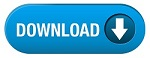Home » SSC » Model Questions for Scientific Assistant (IMD) Exam (Paper-2) PHYSICS – Set 11

# Model Questions for Scientific Assistant (IMD) Exam (Paper-2) PHYSICS – Set 11

## 1. If there is no external torque acting on a system, the angular momentum does not change.

a. Tangential speed
b. Conservation of angular momentum
c. Rotational inertia
d. Torque

## 2. An object that is not being acted on by any forces or torques is in this state.

a. Tangential speed
b. Rotational speed
c. Rotational inertia
d. Equilibrium

## 3. Linear speed experienced by an object that is travelling along a curved path.

a. Tangential speed
b. Rotational speed
c. Rotational inertia
d. Torque

## 4. Tendency of an object to resist a change in its rotation.

a. Tangential speed
b. Rotational speed
c. Rotational inertia
d. Torque

## 5. The number of rotations of an object per unit time.

a. Tangential speed
b. Rotational speed
c. Rotational inertia
d. Torque

### 6. Force multipled by lever arm.

a. Tangential speed
b. Rotational speed
c. Rotational inertia
d. Torque

## 7. The average position, as a single point, through which gravity appears to act on an object.

a. Tangential speed
b. Rotational speed
c. Rotational inertia
d. Center of gravity

## 8. The average position, as a single point, of the mass of an object.

a. Tangential speed
b. Rotational speed
c. Rotational inertia
d. Center of mass

## 9. A farmer replaces the tires on his tractor with smaller diameter tires. For a given RPM, the linear speed of the tractor is now—

a. the same as before
b. more
c. less
d. all of the above

## 10. Based on rotational inertia, which will take longer to roll down an incline… a can filled with ice, or the same can filled with water?

a. ice
b. water
c. both the same
d. depends on the size of the can

#